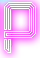# Sum

#### Statement

You are given two numbers A and B. Calculate their sum.

#### Input:

The first line of input contains integers A and B.

#### Output:

Your output should contain only one line, sum of numbers A and B.

#### Constraints:

• 3 ≤ A, B ≤ 109

#### Example input:

``2 3``

#### Example output:

``5``

• 0.1 second
• 4MB